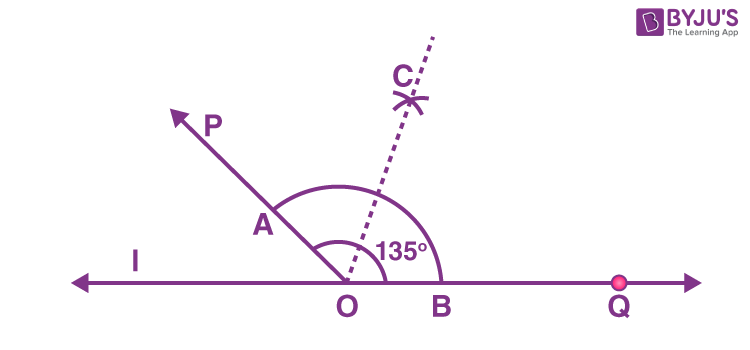# Draw an angle of measure 135° and bisect it.

Steps to construct an angle of measure 135° and its bisector are:
(i) On a line l, using a protractor measure ∠POQ of 135°.
(ii) Draw an arc of convenient radius by taking O as centre. Let this intersect both rays of angle 135° at points A and B, respectively.
(iii) Take A and B as centres, draw arcs of radius more than half of AB in the interior of angle of 135°. Let these intersect each other at C. Join OC.
Thus, OC is the required bisector of angle 135°.(0)(0)